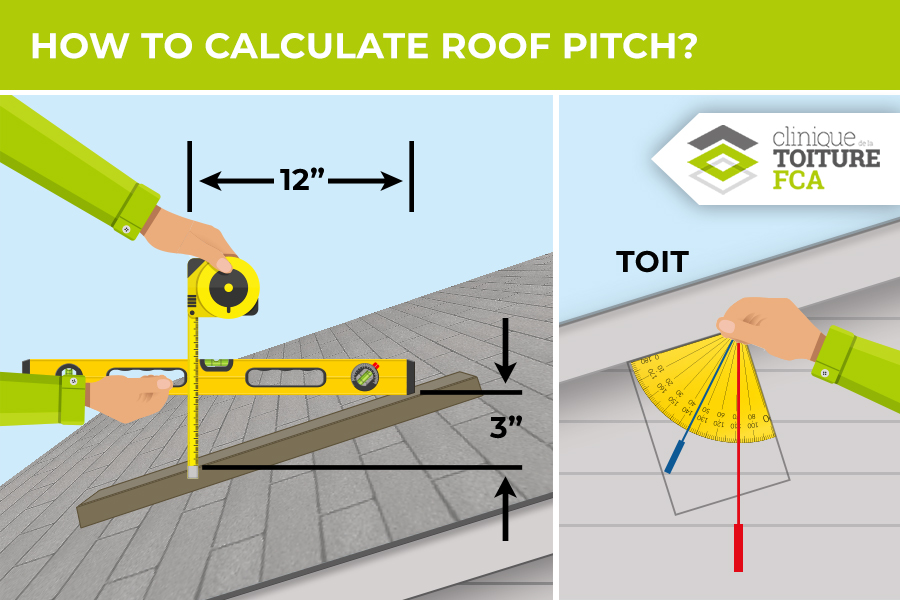# How to calculate roof pitch?

Summary

Whether it’s a simple repair, a major renovation, or even the construction of a new roof, calculating roof pitch is critical for many reasons. Whether you are doing your own renovations or hiring a contractor, knowing the angle of your roof is essential.

## Why calculate the slope of a roof?

If you are planning to work on your roof, no matter how big or small your project is, before you begin you must calculate the roof pitch.

In the case of a new roof, the slope plays a role in the choice of covering: not all materials can withstand a steep slope, and not all are suitable for a flat roof!

If you are doing major renovations, you should also be aware that the slope affects the amount of snow that your roof can support.

Even if you are not doing your own renovations, calculating the roof pitch will help you get more accurate estimates from your contractor. In addition, the contractor will be able to anticipate the safety measures required for their workers.

## Calculating the roof pitch

As you can see, determining the slope of your roof is necessary. But now you are probably asking yourself: how can I calculate the slope of a roof? In truth, there are many ways of doing it. Before we continue, let’s go over the different tools you need and in what unit of measurement you want to use. Do you need to know the angle, the ratio or the percentage?

### Different roof pitch calculations

To calculate the pitch of your roof in percent or degrees, you will need two pieces of information: the height and horizontal width of one side of your roof.

If you want to know the angle as a percentage, simply use the following mathematical formula: P = 100*H/W.

P is the percentage, and H and W are the height and width respectively, which you have measured beforehand.

If you want to get a result in degrees, use the following formula: P = 180*ATAN(H/W)/π. ATAN represents the tangent.

Finally, you may need to get the result in the form of a ratio. The tools you will need for this are a level, a flat piece of wood and a tape measure. Place the piece of wood parallel to the edge of the roof, and the level perfectly horizontal. From the point of contact between these two tools, you will measure a distance of 12 inches: from this point, you will measure the vertical distance between the level and the piece of wood.

The resulting measurement is the first number of the ratio, and the second number will be 12. For example, if you measured a vertical distance of 4 inches, the ratio would be 4:12. A carpenter’s square can also be used to calculate the ratio.

If you need to calculate the pitch as a percentage, you can simply use the following formula: P = (height/ horizontal distance)*100.

If you’re not sure how to do these calculations, there are several specialized computer programs that can help you.## Calculating the slope of a flat roof

Even with flat roofs, there is no such thing as a perfectly flat roof; there is at least a slight incline.

Why is that? Because water would simply collect there. In order for rainwater to drain away, roof builders must give it a certain slope that leads water towards the drain. Flat roofs can have a slope ranging from 1 to 5%, depending on their surface area; the larger the surface area, the steeper the slope.

So the same calculations from the previous section apply!

In short, regardless of the type of work you want to do on your roof and whatever type of roof you have, calculating roof pitch will be an essential step. Clinique de la toiture FCA can help with anything you need, so don’t hesitate to contact us.

## Contact La Clinique de la toiture FCA for any question you may have about calculating roof pitch

Do you need help calculating your roof pitch? La Clinique de la toiture FCA is a team of experts that is always here to lend a helping hand! We can also calculate your roof pitch for you, and give you information about any other project. Take note that we specialize in flat roofs only.